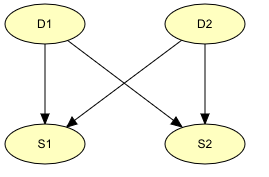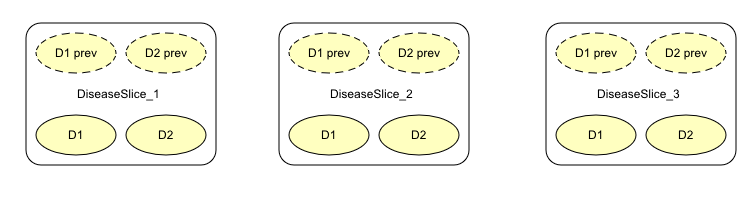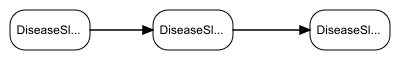# How to Build an Object-Oriented Bayesian Network¶

This tutorial shows how to implement a small object-oriented network in the HUGIN Graphical User Interface. The network we are about to construct is the one modeled in the Diseases example in the Object Orientation tutorial. The qualitative (or structural) representation of our object-oriented network is shown in Figure 1. In this tutorial, we shall ignore the specification of the CPTs.

If you want to understand the design of this object-oriented network, you should read about it in the Object Orientation tutorial. Were we to construct this time-sliced network as a Bayesian network, we would get the network shown in Figure 2.

## Creating the Time-Slice Model¶

A network (i.e., Bayesian network or LIMID) is a special case of an object-oriented network. What makes a network object oriented is the existence of instance nodes (i.e., nodes that represent instances of other networks). Thus, we start out by creating a new empty network by selecting the “New” menu item in the “File” menu. This gives us a new network window containing an empty network called “unnamed<x>”, where x is some integer. It starts up in Edit Mode which allows us to start constructing the object-oriented network immediately (the other main mode is Run Mode which allows you to use the network). ,

In Figure 2, we observe that each of the three time slices contains four nodes: D1, D2, S1, and S2, where D1 and D2 represent two different diseases with states “Present” and “Absent”, and S1 and S2 represent symptoms that both may be observed as consequences of each of the diseases. We shall assume that S1 and S2 represent symptoms with two possible outcomes, “Observed” and “Unobserved”. As each of the time slices are identical, both at the qualitative (or structural) level and quantitative level (i.e., the CPTs are identical, including those that describe the temporal aspect, namely P(D1_2|D1_1), P(D2_2|D2_1), etc.), we need only construct a model describing a generic time slice and then connect three instances of this network.

### Creating the Nodes¶

First, we construct the generic time-slice model, containing the four nodes D1, D2, S1, and S2. The nodes all represent discrete chance variables. Therefore, we select the Discrete Chance Tool and create the four nodes by clicking the left mouse button at four different locations in the network pane while keeping the Shift key down (to avoid reselecting the tool for each node). We then change the default names of the nodes and their default state names using the Node Properties pane. Second, we select the Link Tool and create the link from D1 to S1 by dragging the mouse cursor (i.e., pressing the left mouse button and moving the mouse cursor while keeping the button pressed) from a point inside D1 to a point inside S1 and then releasing the mouse button. Again, we keep the Shift key pressed, and create the other three links in the same manner. The result is illustrated in Figure 3.Figure 3: BN for a single time slice of the Diseases problem.¶

### Output Nodes¶

Now, in order for a network for a single time-slice have parent nodes in the immediately preceding network, we need to be able to refer to nodes outside the network in Figure 3. In a conventional BN, this is not possible. Thus, as D1 and D2 are going to be parents of D1 and D2, respectively, in the next time slice, we must declare D1 and D2 as output nodes, making them visible outside the network (or rather through instances of the network).

### Input Nodes¶

Also, in the network in Figure 3, we should be able to specify the temporal aspect, namely the CPTs P(D1|D1 prev) and P(D2|D2 prev), where the nodes “D1 prev” and “D2 prev” are placeholder nodes for D1 and D2, respectively, in the immediately preceding time slice. Such placeholder nodes are referred to as input nodes, and shouldn’t be confused with real nodes. A real node, which is type consistent with an input node, can be bound to that input node. That is, an input node becomes identical with the node that is bound to it. However, if an input node hasn’t got a binding associated with it, the network containing the input node can still be used (i.e., compiled in to a junction tree and used for inference). In that case the input node is treated as a real node. That is, each input node has a CPT associated with it just as any ordinary node, but this CPT is used only if no nodes have been bound to the input node in a network containing an instance of the network in which the input node is defined. Input nodes and output nodes are collectively referred to as interface nodes.

### Creating the Interface Nodes¶

Now, let’s try to put all this into practice. First, we declare D1 and D2 as output nodes. This is done by clicking the “Output” check box in the Node Properties pane for each of them. To indicate their new status as output nodes, D1 and D2 now are drawn with thick borders of the color selected for interface nodes (as set in the Network Properties pane).

To create the two input nodes, “D1 prev” and “D2 prev”, we first create two ordinary nodes and set their names to D1_prev and D2_prev (and/or their labels to “D1 prev” and “D2 prev”), respectively, in the Node Properties pane. Also, in the Node Properties pane for each of these two new nodes, we click the “Input” check boxes to declare them as input nodes. Similar to D1 and D2, “D1 prev” and “D2 prev” are drawn with thick borders of the interface nodes color. In addition, the appearance of the (regular) borders of “D1 prev” and “D2 prev” changes from solid to dashed, which indicates that they are not real nodes.

Finally, we create links from “D1 prev” and “D2 prev” to D1 and D2, respectively. The result of these operations appear in Figure 4.Figure 4: BN for a single time slice of the Diseases problem, including specifications of interface nodes.¶

## Creating the Diseases Mode¶

To create the final Diseases model spanning three time slices, we first create a new empty network (via the “New” menu item in the “File” menu). Next, we select the Instance Tool and create three instance nodes by clicking the left mouse button at three different locations in the network pane while keeping the Shift key down. The result appears in Figure 5 (scaled to 81%).Figure 5: The Network Pane contains the three instance nodes that represent three instances of the time-slice model shown in Figure 4.¶

Each instance node appear as a rectangle with rounded corners. We note that the nodes declared as interface nodes in the generic time-slice model appear in each of the instance nodes. The input nodes appear in a row at the top of the instance node, and, similarly, the output nodes appear at the bottom of the instance node.

Next, we need to bind the output nodes of instances DiseasesSlice_1 and DiseasesSlice_2 to the input nodes of instances DiseasesSlice_2 and DiseasesSlice_3, respectively. This is done by creating links (via the :ref:` Link Tool<Link_tool>` ) from the output nodes to the corresponding input nodes. The resulting model appears in Figure 6.

Obviously, the model would look nicer if the order of appearance of the input nodes were reversed. If we select the Select Tool, we can easily alter the order of appearance of the interface nodes. We move an interface node one position to the left (right if the Shift key is down) by placing the mouse cursor on top of the interface node and clicking the left mouse button. After reordering the network appears as in Figure 7.Figure 7: The order of appearance of interface nodes can be altered through simple mouse clicks..¶

Finally, it is often desireable to be able to collapse one or more of the interface nodes in order to hide away irrelevant details, thereby making the network much less cluttered. Again, if we activate the Select Tool, we can collapse (expand) an expanded (collapsed) instance node, simply by clicking the left mouse button right outside the node. Alternatively, we can choose the “Collapse Instance Nodes” (“Expand Instance Nodes”) menu item in the “View” menu, which collapses (expands) all instance nodes.

The object-oriented network in Figure 7 with the instance nodes collapsed appear in Figure 8.Figure 8: The object-oriented network in Figure 7 with the instance nodes collapsed.¶

## Compiling the Object-Oriented Network¶

Now, assuming the CPTs of the time-slice model in Figure 4 has been filled in (see the tutorial on BNs for details), it is the time to compile the network and see how it works:

• Press the Run Mode tool button in the Tool Bar (see Figure 9).

• For each configuration of parent states in the CPT of a node the probabilities of the different states of the node must sum to 1. In other words, each column of the table must sum to 1. If there is a column that does not sum to 1, the compiler will normalize the values. This fact can be utilized when filling in the probabilities. Say, for example, that the probability of D1=Present in the first time slice is based on the observation of 13527 patients, 168 of whom were observed to have the disease. Instead of first computing the fractions, you just put 168 in the Present state of D1, and 13359 in the Absent state. Then the compiler will compute the proper values.

The compilation of small networks like the Diseases network is completed in very short time. After the compilation, the Run Mode is entered (we have so far only been working in Edit Mode).

## Running the Object-Oriented Network¶

In Run Mode, the network window is split into two by a vertical bar (see Figure 10). To the left is the Node List Pane and to the right is the Network Pane.Figure 10: The network window in Run Mode. To the left is the Node List Pane (with all nodes collapsed) and to the right is the Network Pane.¶

You can view the probabilities of a node being in a certain state by expanding the node in the Node List Pane. You expand (collapse) a node by clicking its expand (collapse) icon in the Node List Pane, by double-clicking its node symbol in the Node List Pane, or by selecting (deselecting) it in the Network Pane. You can also expand (collapse) all nodes at once by pressing the expand (collapse) node list tool in the Tool Bar just to the right of the node properties tool.

Unlike basic nodes, instance nodes don’t have belief monitors associated with them, as they represent entire (sub)networks. Instead we must expand the instance node, whereby we get to see the list of nodes of the (sub)network that the instance node represents (see Figure 11).Figure 11: The nodes of the network represented by an instance node, get displayed in the Node List Pane when the instance node gets selected.¶

If the instances contains many nodes, locating a given node in the node list may be difficult. When this is the case, the instance can be “traversed” by right-clicking on the node and selecting “Traverse Instance” (or selecting the node and choosing “Traverse Instance” from the network menu). This will open a window with compiled version of the class from which the instance node is created. In this window evidence can be inserted, and then transferred to any available instance. When the instances contains many nodes, it is possible to hide all private nodes from the node list to prevent the list from being to cluttered. This is done by selecting Toggle Private Nodes in the View menu. For more details on the Run Mode, please consult the How to Build BNs tutorial.